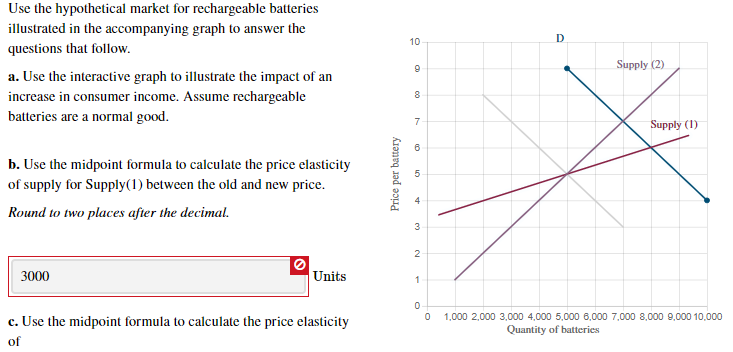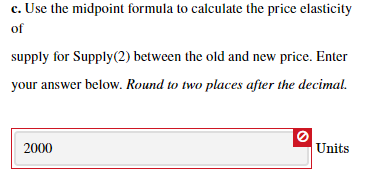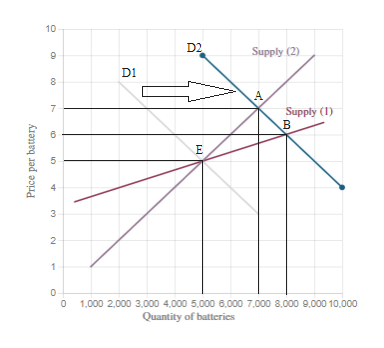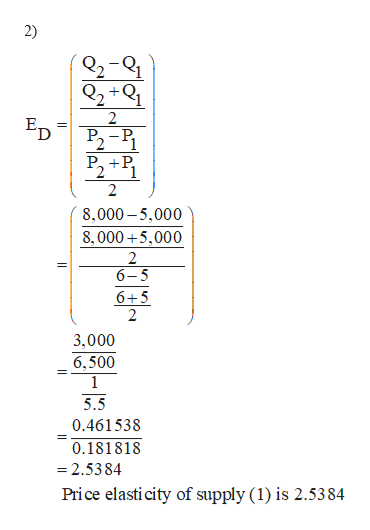# Use the hypothetical market for rechargeable batteriesillustrated in the accompanying graph to answer the10questions that follow.Supply (2)a. Use the interactive graph to illustrate the impact of anincrease in consumer income. Assume rechargeablebatteries are a normal good.Supply (1)вb. Use the midpoint formula to calculate the price elasticityof supply for Supply(1) between the old and new price.Round to two places after the decimal.3000Units1,000 2,000 3,000 4,000 5,000 6,000 7,000 8,000 9,000 10,000c. Use the midpoint formula to calculate the price elasticityQuantity of batteriesofPrice per battery c. Use the midpoint formula to calculate the price elasticityofsupply for Supply(2) between the old and new price. Enteryour answer below. Round to two places after the decimal.2000Units

Question
55 viewshelp_outlineImage TranscriptioncloseUse the hypothetical market for rechargeable batteries illustrated in the accompanying graph to answer the 10 questions that follow. Supply (2) a. Use the interactive graph to illustrate the impact of an increase in consumer income. Assume rechargeable batteries are a normal good. Supply (1) в b. Use the midpoint formula to calculate the price elasticity of supply for Supply(1) between the old and new price. Round to two places after the decimal. 3000 Units 1,000 2,000 3,000 4,000 5,000 6,000 7,000 8,000 9,000 10,000 c. Use the midpoint formula to calculate the price elasticity Quantity of batteries of Price per battery fullscreenhelp_outlineImage Transcriptionclosec. Use the midpoint formula to calculate the price elasticity of supply for Supply(2) between the old and new price. Enter your answer below. Round to two places after the decimal. 2000 Units fullscreen
check_circle

Step 1

a)

The increase in income of the consumer increases the quantity demanded for goods. Thus, it shifts the demand curve to rightward from D1 to D2. “E” is the initial equilibrium point in the market. The shift in demand curve leads to the movement of equilibrium point from “E” to “A” on supply curve (2) and from “E” to “B” on supply curve (1). Figure is shown below.help_outlineImage Transcriptionclose10 D2 Supply (2) D1 Supply (1) в 1,000 2,000 3,000 4,000 5,000 6.000 7,000 8,000 9,000 10,000 Quantity of batteries Price per battery 3. fullscreen
Step 2help_outlineImage Transcriptionclose2) 2-Q1 Q2 +Q1 ED P2-P P, +P, 8,000 – 5,000 8, 000 +5,000 2 6-5 6+5 3,000 6,500 5.5 0.461538 0.181818 = 2.5384 Price elasti city of supply (1) is 2.5384 fullscreen

### Want to see the full answer?

See Solution

#### Want to see this answer and more?

Solutions are written by subject experts who are available 24/7. Questions are typically answered within 1 hour.*

See Solution
*Response times may vary by subject and question.
Tagged in